Phase Controlled Rectifiers or Converter

# Phase Controlled Rectifiers or Converter - Notes | Study Electrical Engineering SSC JE (Technical) - Electrical Engineering (EE)

 1 Crore+ students have signed up on EduRev. Have you?

Phase Controlled Rectifiers or Converter

Phase Controlled Rectifiers or Converter Generation and transmission of electrical energy is through alternating current and voltage. Main utilization is also in AC form. However, some special applications require DC. Some such applications are electrochemical processes, DC traction systems, high voltage DC transmission systems etc.

The conversion of AC to DC is known as rectification. The rectifier circuits comprise of either diodes or thyristor. Diode rectifiers are known as uncontrolled rectifier because for a fixed value of AC input voltage, the output DC voltage is fixed and connot be changed. P?or some low power devices where control or output voltage is not required, rectifier circuits using diodes are used. Such circuits have the advantage of low cost and simple circuitry.

Rectifier circuit using thyristors are known as controlled rectifier. This is evidently, due to the reason that by changing the firing angle of thyristor the output DC voltage can be controlled. Since thyristors are available in high voltage, high current ratings, controlled rectifiers (using thyristors) are used in many high power devices.

A controlled rectifier may be single phase or 3 phase circuit depending on whether the input is single phase or 3 phase AC. High power circuits are all 3 phase devices. Each oi” these may be semi- converter, full converter or dual converter. A rectifier is also known as AC-DC converter.

A semiconverter is a one quadrant converter. It has one polarity of output voltage and current a full converter is a two quadrant converter. The polarity of its output voltage can be either positive or negative.
However, its output current has one polarity only. A dual converter is a 4 quadrant converter and both its output voltage and current can be either positive or negative.

Single Phase Half-Wave Converter The circuit arrangement for the rectifier in shown in figure. The output is varied by varying the firing angle a of the thyristor. The diode D (shown by dotted line) in called free wheeling or commutating diode. It prevents reversal of load voltage except for small diode voltage drop and transfer the load current away from the rectifier, thereby allowing the thyristor to regain its blocking state.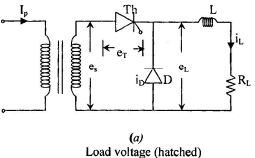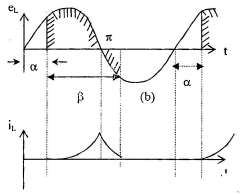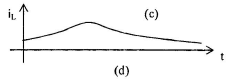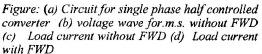Various current and voltage waveforms are shown in figue. The conduction of the thyristor starts at α and ceases at β ( »p ). Because of the inductance in the circuit, the conduction does not stop at π, but extends upto an angle ø of the negative cycle.This causes a negative voltage flow in the load as shown.
The load current is shown in the second waveform. If the freewheeling diode (FWD) is incorporated in the circuit, its conduction  starts immediatedly at π and neutralizes e.m.f. induced in the inductance and enables the thyristor to recover. The load current decays exponentially but not below holding current.

Circuit Analysis

Period I : α < ωt (SCR conducts)

Period II : α < ωt < 2π + α (FWD conducts)
Average voltage across the load is given by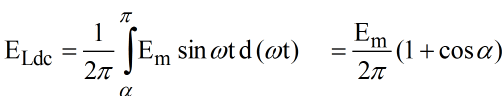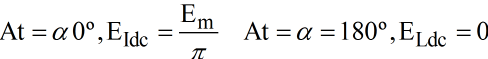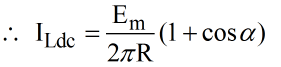(for resistive load)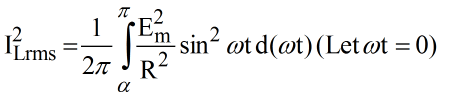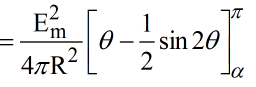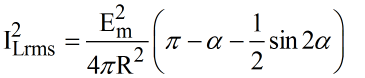The advantages of using freewheeling diode are:

1. Improvement of waveform of load current
2. Improvement of power factor
3. Improvement of circuit performance

Signal Phase Full Wave Converter

As seen from the figure two thyristors T1 and T2 are used and each one conducts alternately, while the other is reverse biased. A center tapped transformer is used. When T1 is tuned on at a, it conducts upto (π + α) on account of load inductance. At this instant T2 gets tuned on. With a free wheeling diode, the T1 conducts only for a to π instead toa to (π + α)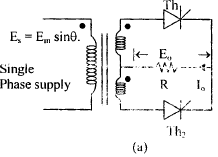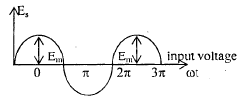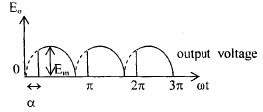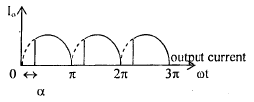Average votlage without free wheeling diode is ,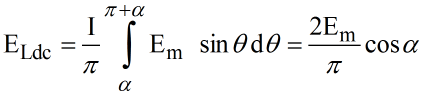Hence,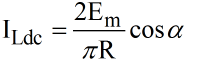Average voltage with free wheeling diode is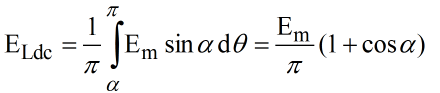and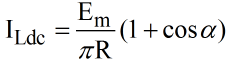If the inductance in supply circuit is assumed to be zero the transfer of load current from T1 to T2 takes place instantaneously. A finite value of inductance in the supply circuit causes a delay in the transfer of current from one thyrsitor to the other. These conduct load current simultaneously for a small amount of time called overlap and the corresponding angle is called overlap angle. The tansfer of current is called commutation. During the commutation load voltage is zero in the converter.

Single phase fally - controlled bridge converter

The circuit is shown in figure (a) and voltage and current waveform’s in figure (b) and (c) respectively.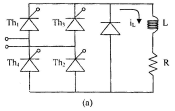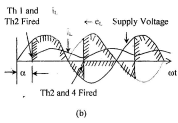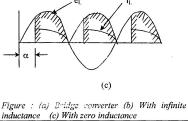Thyristor 1 and 2 are fired at an angle a in the positive half cycle and thyristor 3 and 4 are fired in negative half-cycle.

Output votlage is obtained as shown in figure (b) and (c). In figure (b) load inductances is assumed to be infinite, so a smooth direct current is obtained. The effect of presence of inductance in the circuit is to make the current unidirectional even when the input voltage, polarity is reversed, current continues to flow through Th 1 and 2 till the other pair Th 3 and 4 as fired symmetrically at angle a in negative half cycle. When Th 3 and 4 are fired, Th 1 and 2 are revered biased and turn-off. Load current flows through Th 3 and 4. When there is no inductance in the circuit, output voltage remain positive only and load current reaches zero at the end of every half cycle. There is no current from the end of halfcycle to the instant where other pair of thyristors is fired. The input current is alternating

Average Output DC voltage is given by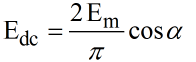(without FWD)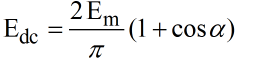(without FWD)

From this expression it is clear that load voltage is negative when α > 90º. This operation involves returning of DC power to the source and the rectifier is said to work as an inverter.

Single Phase Half-Controlled Bridge Converter The circuit arrangement for the half-controlled bridge rectifier is shown in figure. Here two thyristors and two diodes are used, these diode also serve as free-wheeling diode.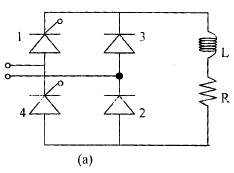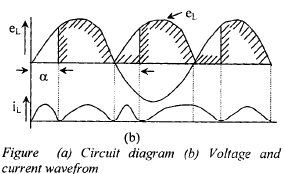Average output voltage is given by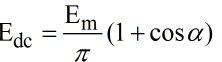Effect of source inductance

When the source inductance is present, thyristors conducting in first half-cycle (say 1 and 2) do not turns off immediately after thyristor in later half- cycle (3 and 4) are fired. The current continues to flow through 1 and 2 even though polarity of applied voltage is reverse and current gradually shifts to 3 and 4. This duration is known as overlap period,- during this output voltage is zero. The angle corresponding to overlap period is called overlap angle and is denoted by y explains the effect of source inductance.

A.C. line Current In full wave rectifier operation large reactive power flow is needed. This increases with increase in firing angle. Free wheeling diodes improve the input power factor and average DC output voltage. The AC line current flows in one direction when the angle varies from α to π and in opposite direction when angle varies from (π + α) to 2π. The phase angle of the fundamental component of input current is less than a and input reacti ve pow er i s reduced. Phase angle (ø) is a v ithout free wheeling diode and is [a / 2] with free wheeling diode.
Fundamental r.m.s. value of AC line current is given by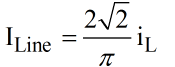(Without FWD)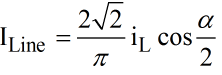(With FWD)

Dual converter 

• To obtained four quadrant operation, two full converter (1 - f or 3 -f) are connected back to back across the load. 
• So to avoid circulating current a a1 + a2= 180º . 
• It is seen that even when a1 + a= 180º , there is circulating current because, with the condition a1 + a= 180º the average voltage of the two converters V01, and V02 are equal but their instantaneous values will still not same. 
• Further to reduce the circuiting currentinterphase reactor is introduced (function of this reactor is some as discussed in parallel operation of thyristor) in the circuit).

Comparison of Circulating Current Mode and Non-Circulating Current Mode Dual Converter

A dual converter is always designed and operated in circulating current mode. The relative advantages and disadvantages of the two mode of operation are as under:

1. In non-circulating current mode only one of the converters operates at one time. However in circulating current mode both the converters operated simultaneously. .

2. In non-circulating current mode, the system operates in discontinuous mode. Before the firing pulse in applied to the second converter it is necessary that decay of load current supplied by first converter is achieved. A delay of about 10-20 milli seconds between the turning off of one converter and firing of second converter is necessary. In circulating current mode both converts operate simultaneously and hence continuous conduction takes place.

3. Basically a non-circulating current mode dual converter does not need a reactor. However, if the load inductance is very small, a reactor may be needed to make the load current circulating and constant. A circulating current mode dual needs a reactor to limit circulating current. This reactor is costly.

4. In circ ulating current mode one converter operates as rectifier and the other operators as inverter. Therefore, power How can be either direction at any time.

5. In non-circulating current mode the response is slow due to discontinuous current operation.
However in circulating current mode the response is fast.

6. A slight mal-operation in firing scheme may result in dead short-circuit in non-circulating current mode. This may cause heavy short- circuit current.
However in circulating current mode the fault current is limited by the reactor.

7. The non-circulating current mode has lower losses and higher efficiency as compared to circulating current mode.

9. The non-circulating current system is cheaper than the circulating current system.

10. The non-circulating mode needs a command module to sense the change in polarity. However, both converters are in simultaneous operation in the circulating current mode and hence no such command module is required.

The document Phase Controlled Rectifiers or Converter - Notes | Study Electrical Engineering SSC JE (Technical) - Electrical Engineering (EE) is a part of the Electrical Engineering (EE) Course Electrical Engineering SSC JE (Technical).
All you need of Electrical Engineering (EE) at this link: Electrical Engineering (EE)

## Electrical Engineering SSC JE (Technical)

2 videos|75 docs|37 tests

## Electrical Engineering SSC JE (Technical)

2 videos|75 docs|37 tests

Track your progress, build streaks, highlight & save important lessons and more!

,

,

,

,

,

,

,

,

,

,

,

,

,

,

,

,

,

,

,

,

,

;